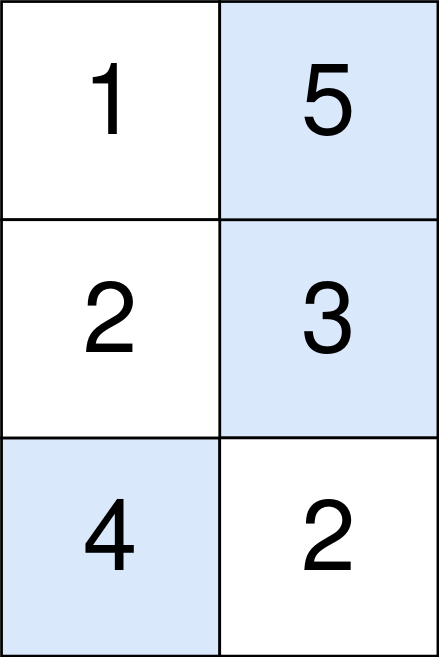# 扣分后的最大得分

## 1937. 扣分后的最大得分 (Medium)

`abs(x)` 定义为：

• 如果 `x >= 0` ，那么值为 `x` 。
• 如果 `x < 0` ，那么值为 `-x` 。```输入：points = [[1,2,3],[1,5,1],[3,1,1]]

``````输入：points = [[1,5],[2,3],[4,2]]

```

• `m == points.length`
• `n == points[r].length`
• `1 <= m, n <= 105`
• `1 <= m * n <= 105`
• `0 <= points[r][c] <= 105`